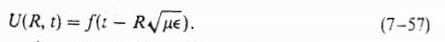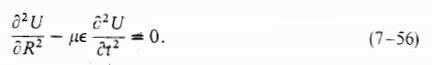# Need help proving that a function is a solution to the homogeneous wave equation

JerryG

## Homework Statement

I have a homework problem that says that any function of the below form is a solution to the homogeneous wave equation.Any function of this form is a solution to the following equation:I would be able to solve it if the function was defined, but I'm not quite sure how to handling the partial differential equations using this general solution.

Let $$y = t -R\sqrt{\mu\epsilon}$$ and use the chain rule on $$U(R,t) = f(y)$$.